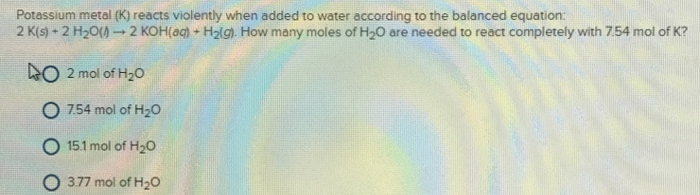# Problem: Potassium metal (K) reacts violently when added to water according to the balanced equation: 2K(s) + 2 H2O(l) → 2 KOH(aq) + H2(g). How many moles of H2O are needed to react completely with 754 mol of K? a. 2 mol of H2O b. 7.54 mol of H2O c. 15.1 mol of H2O d. 3.77 mol of H2O

###### FREE Expert Solution
83% (212 ratings)###### Problem Details

Potassium metal (K) reacts violently when added to water according to the balanced equation:

2K(s) + 2 H2O(l) → 2 KOH(aq) + H2(g). How many moles of H2O are needed to react completely with 754 mol of K?

a. 2 mol of H2

b. 7.54 mol of H2

c. 15.1 mol of H2

d. 3.77 mol of H2O